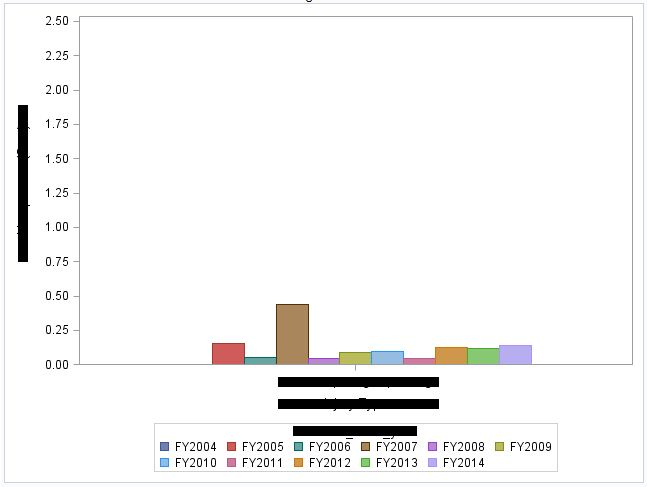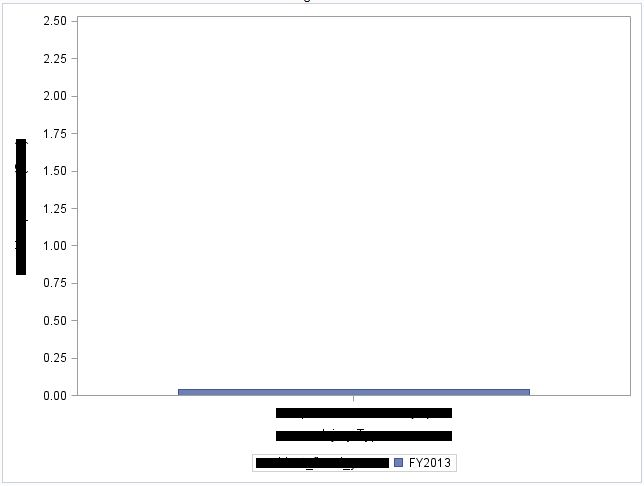## SGPLOT legend doesn't show empty groups

Hello all,

I am currently writing a quick macro to compute bar charts. Each bar chart corresponds to a specific type within a categorical variable, with a bar for each year and the response being the rate of this type occurrence in said year, relative to the population. I have almost everything plotting how I want it, but for consistency, I want the legend to include the year even if the rate was zero for that year. For example, there are 11 years total, but one of the types only occurs in 2013, so the plot shows just one, really wide, bar and the legend only shows year 2013. I want the bar to be scaled to match the size of a bar in a type that occurs every year and I want the legend to list all 11 years.

My code so far is;

%macro plots (val=);

proc sgplot data=ABC (where=(X="&val"));

vbar X/group=YEAR response=RATE

groupdisplay=cluster grouporder=ascending;

yaxis values=(0 to 2.5 by 0.25);

run;

%mend plots;

Here are some images.Thanks everyone!

1 ACCEPTED SOLUTION

Accepted SolutionsDanH_sas
SAS Super FREQ

## Re: SGPLOT legend doesn't show empty groups

Given your scenario, I would create a data step where I would iterate by each value of X and have a "year" loop to init the "response" column to 0. Then, use PROC APPEND to append the real data set to the end of that data set. The result should create entries for all combinations of category and group such that the bars and legend behave as you requested without affecting the original data calculations in VBAR. GIve that a try and let me know if it works out for you.

Thanks!

Dan

2 REPLIES 2DanH_sas
SAS Super FREQ

## Re: SGPLOT legend doesn't show empty groups

Given your scenario, I would create a data step where I would iterate by each value of X and have a "year" loop to init the "response" column to 0. Then, use PROC APPEND to append the real data set to the end of that data set. The result should create entries for all combinations of category and group such that the bars and legend behave as you requested without affecting the original data calculations in VBAR. GIve that a try and let me know if it works out for you.

Thanks!

Dan

## Re: SGPLOT legend doesn't show empty groups

Thank you Dan!!

Although I did not use your method, you pointed out my mistake. I used proc freq to output the data set and assumed 0 counts would be included in this set. I went back and used the SPARSE option in proc freq to include these 0's, effectively doing what you suggested.

Thanks so much for the quick response!

Discussion stats
• 2 replies
• 1708 views
• 1 like
• 2 in conversation